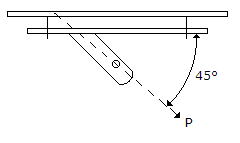# Civil Engineering - UPSC Civil Service Exam Questions

46.

A clay soil specimen when tested in unconfined condition gave an unconfined compressive strength of 100 kN/m2. A specimen of the same clay with the same initial condition is subjected to a UU triaxial test under a cell pressure of 100 kN/m2. The axial stress (in kN/m2) at failure would be

 A. 150 B. 200 C. 250 D. 300

Explanation:

No answer description available for this question. Let us discuss.

47.

A propped cantilever of span 4 m is fixed at A and propped at B. The beam carries a u.d.l. of 1 t/m over the entire span. The reaction at B is

 A. 5/2 t B. 2 t C. 1 t D. 3/2 t

Explanation:

No answer description available for this question. Let us discuss.

48.

The spacing of tile drains to relieve water-logged land is directly proportional to the

 A. depth of drain below the ground surface B. depth of impervious strata from the drain C. depth of drain below the water level D. coefficient of permeability of the soil to be drained

Explanation:

No answer description available for this question. Let us discuss.

49.

Four bolts share the load P as shown in the figure. The shearc strength of bolt is 30 kN and tension strength of bolt is 40 kN. Which one of the following is the value of P ?A. 96 kN B. 105 kN C. 117 kN D. 134 kN

Explanation:

No answer description available for this question. Let us discuss.

50.

The object of chain and cross-staff survey is to
1. locate the boundaries of an area.
2. plot the figure to a scale.
3. find the area of the plot.
4. find the reduced levels of the plot.
Which of the above statements is/are correct ?

 A. 1, 2, 3 and 4 B. 1, 2 and 3 C. 1 and 2 D. 4 alone